The Singing Hedgehog Guide to:
Long Division 3

Here is 552 ÷ 16 in small stages to make the method clear.

It is often useful to start by jotting down the relevant table, in this case the sixteens.
Note the cunning way I have set them out:

2 x 16 = 32            3 x 16 = 48            5 x 16 = 80
4 x 16 = 64            6 x 16 = 96           [2 x 16 = 32]
8 x 16 = 128          9 x 16 = 144          7 x 16 = 112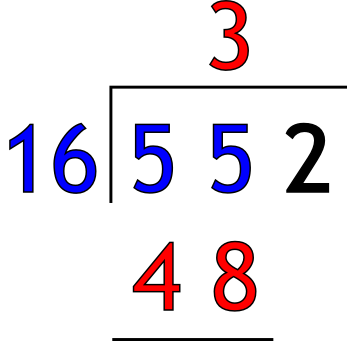Set out the sum and do the first division.

16 into 55 goes 3 times
3 x 16 is 48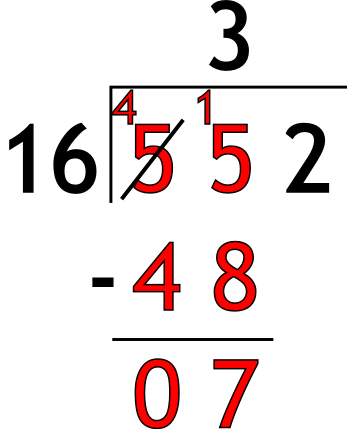Do the subtraction.

55 - 48 = 7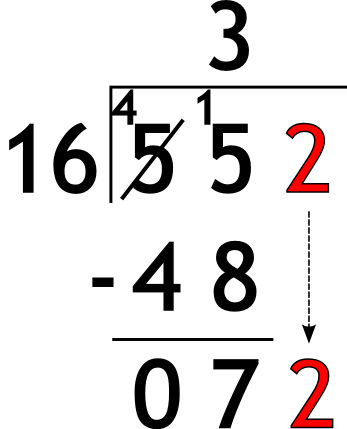Bring down the next digit.

bring down 2 to make 72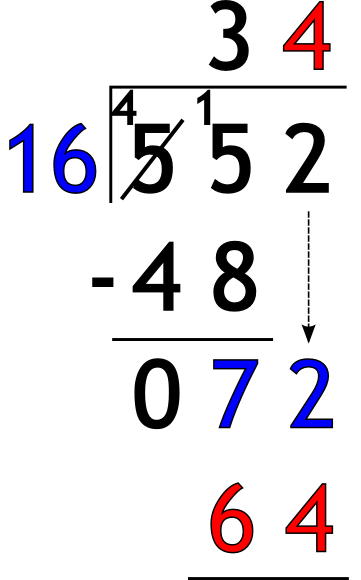Do the next division.

16 into 72 goes 4 times
4 x 16 is 64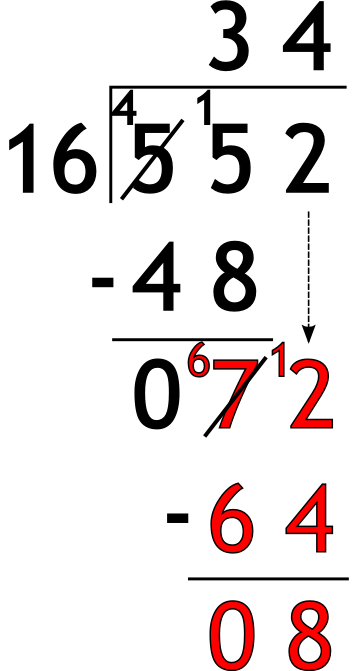Do the subtraction.

72 - 64 = 8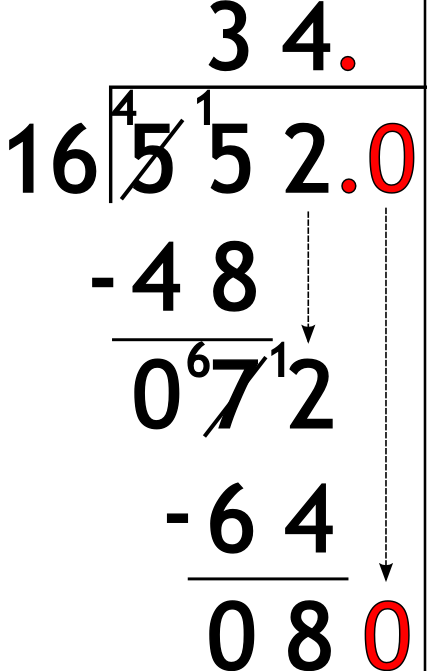Bring down the next digit.

There is no digit written so we create one!
Pop in a pair of decimal points, fill in a zero
bring down 0 to make 80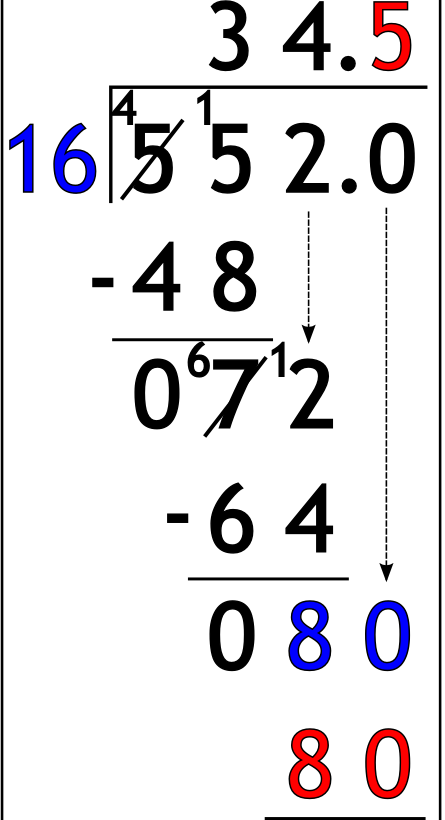Do the next division.

16 into 80 goes 5 times
5 x 16 is 80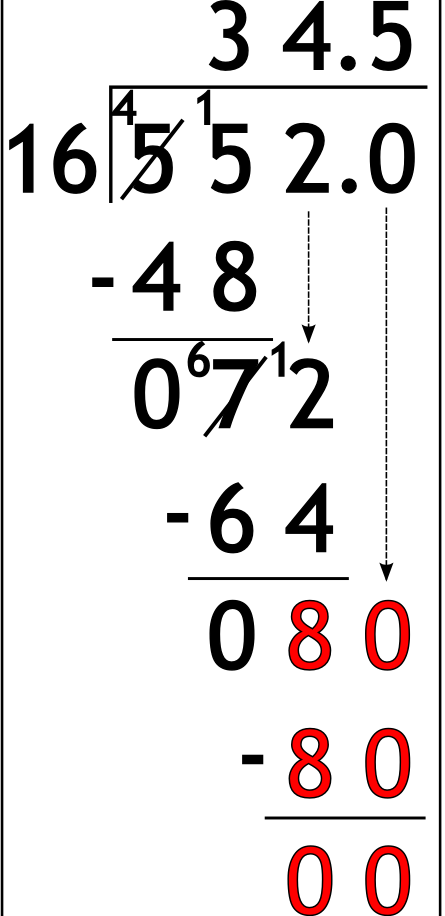Do the subtraction.

80 - 80 = 0

finished!

Now you need to practise: